## 7 Ways to Show Formulas in Excel (Easy Step-by-Step Guide)

Excel formulas are essential for reports, forecasts, and data analysis. Displaying formulas in Excel can help you better understand your data. For instance, if you need to print a worksheet with its formulas, displaying them is crucial. In this guide, we'll walk you through seven easy methods for revealing the formulas behind your data, empowering you to work with confidence and efficiency.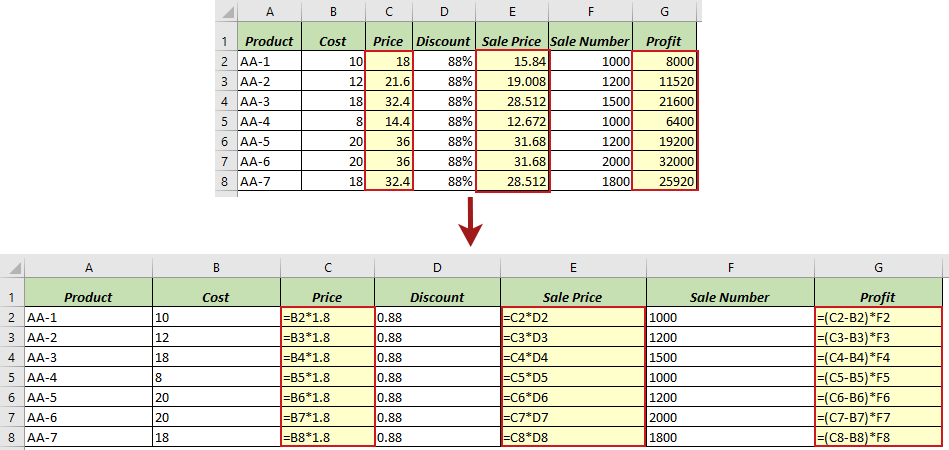### Show all formulas in a sheet

In this case, there are formulas in Columns C, E, and G in the worksheet below, and we want to display all the formulas in the sheet.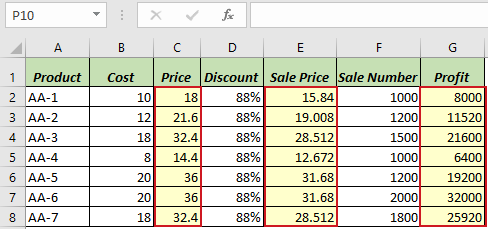Excel offers multiple methods for revealing all the formulas in a worksheet. In this section, we'll explore four methods to achieve this goal.

Method Range Notes
Show Formulas command Current sheet Quickly toggle on or off.
Kutools for Excel Current sheet or all sheets Effective with added features.
Ctrl + ` shortcut Current sheet Quickly toggle on or off; Instant.
Excel Options Current sheet Requires multiple steps.

#### Using Show Formulas command in the ribbon to show all formulas in a sheet

The Show Formulas command allows users to quickly switch between displaying all formulas and their results within the current sheet.

##### Step 1: Toggle on Show Formulas command

In your Excel worksheet, navigate to the Formulas tab. Click on the Show Formulas button in the Formula Auditing group.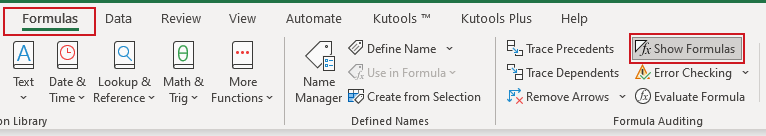##### Result

All formulas in the specified worksheet will now be visible.Tip: To hide all formulas and show the results in the sheet, click on the Show Formulas button again to toggle it off.

#### Using Kutools to easily display all formulas in one or all sheets

Kutools for Excel presents its robust View Options feature, which goes beyond Excel's conventional formula display capabilities. While Excel traditionally allows for formula visibility on a single sheet at once, Kutools gives users the flexibility to easily reveal formulas either on an individual sheet or across the entire workbook. In addition to formula insights, this feature smoothly integrates settings such as Inner Tabs, Formula Bar, and Status Bar, enhancing your workflow and elevating your overall Excel experience.

After installing Kutools for Excel, select Kutools > Views > View Options to open the View Options dialog box. Then please do as follows:

1. Check the Formulas checkbox in the Window options section.
2. Click the Apply to all sheets button for showing all formulas in all sheets in a workbook. ( Skip this step if you only want to show formulas in current sheet.)
3. Click OK.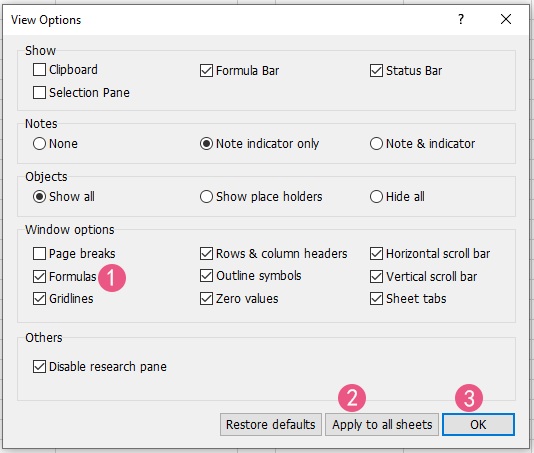Tips:
• To use this feature, you should install Kutools for Excel first, please click to download and have a 30-day free trial now.
• To hide all formulas and show the results in one or all sheets, uncheck the Formulas checkbox in the View Options dialog.

#### Using Ctrl + ` shortcut to show all formulas in a sheet

You can also use the Show Formulas shortcut to toggle between displaying formulas and their results in a sheet.

In your Excel worksheet, simply press Ctrl + `. Now all formulas in the current sheet will be visible.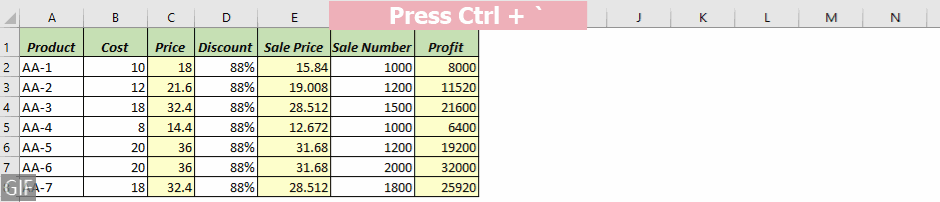Tips:
• The grave accent key (`) is located at the far left of the row containing the number keys, adjacent to the number 1 key.
•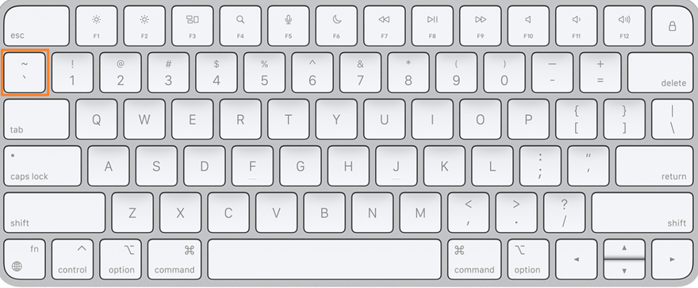• To hide all formulas and show the results in the sheet, press Ctrl + ` again.

#### Using Excel Options to show all formulas in a sheet

Another way to display formulas in a worksheet is by adjusting Excel's settings through Excel Options .

##### Step 1: Open the Excel Options dialog

For Excel 2010 and later versions, navigate to File > Options. For Excel 2007, click the Office Button, then choose Excel Options.

##### Step 2: Specify the settings in the Excel Options dialog:
1. Select Advanced on the left-hand side.
2. Scroll down to the Display options for this worksheet section, and select the specific sheet where you want to show formulas.
3. Check the Show formulas in cells instead of their calculated results checkbox.
4. Click OK.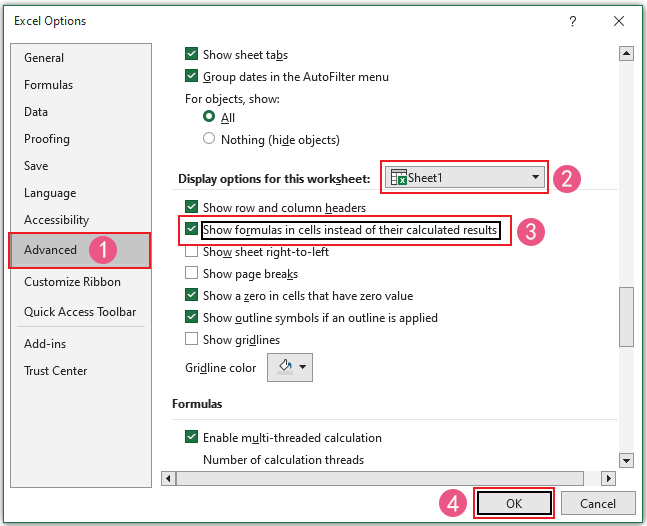##### Result

All formulas in the worksheet you specified will now be visible.Tip: To hide all formulas and show the results in the sheet, uncheck the Show formulas in cells instead of their calculated results checkbox in the Excel Options dialog.

##### Important things you should know:
• No matter which method you select from the four methods mentioned above for displaying formulas in a worksheet, all of them essentially activate the Show Formulas mode.
If the Show Formulas button under the Formula tab is toggled on, it means the Show Formulas mode is activated.
•• When the Show Formulas mode is activated in Excel, columns might temporarily expand to display longer formula text. Don't worry, columns will automatically restore their original widths once the Show Formulas mode is deactivated.
• After the Show Formulas mode is activated in Excel, the Undo button will not work if you wish to revert to the original state.

### Show formulas in selected cells

At times, we may prefer not to display all formulas in a sheet, but only those within selected cells. For instance, there are formulas in Columns C, E, and G in the worksheet below, and we only wish to reveal the formulas in cells G2:G8 of Column G.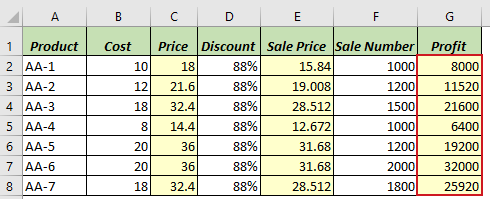In this section, We'll delve into three methods to accomplish this goal.

#### Using FORMULATEXT function to show formulas in selected cells

The Excel FORMULATEXT function retrieves a formula from a specified reference and returns it as a text string. You can use this function to extract and display the formula content of a cell as text

Note: The FORMULATEXT function is only available in Excel 2013 and later versions.

##### Step 1: Apply the FORMULATEXT formula

Click on an empty cell; for this example, I'll choose cell H2. Then type the below formula and press Enter key to retrieve the formula from cell G2.

``=FORMULATEXT(G2)``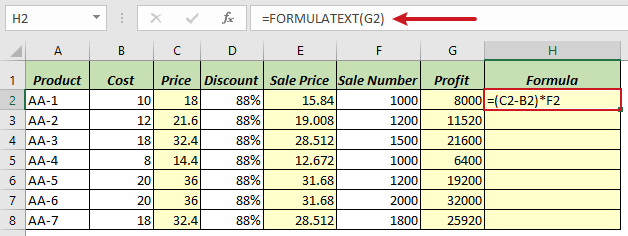##### Step 2: Copy the formula down to below cells to get all results

Double-click on the fill handle of the formula cell to apply the formula to the cells below.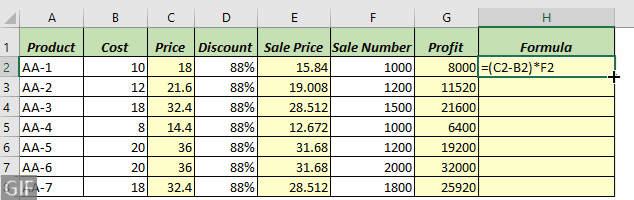#### Using a handy tool to quickly show formulas

For displaying formulas in specific cells, Kutools for Excel's Convert Formula to Text feature allows you to quickly transform formulas into plain text within the same cell range, eliminating the need for extra steps such as creating a helper column when using the FORMULATEXT function. This provides a straightforward way to view formulas in selected cells.

After selecting the cells where you want to show formulas, click Kutools > Conversion > Convert Formula to Text.

Tips:

#### Using Find and Replace feature to show formulas in selected cells

Another method to show formulas in selected cells is using the Find and Replace feature in Excel.

Note: This Find and Replace method isn’t suitable for formulas contains more than one equal sign, such as”=A1=B1”, because it may return the wrong results.

##### Step 1: Select the range of cells where you want to show formulas

Here I select the range G2:G8.##### Step 2: Press Ctrl + H to open the Find and Replace dialog

Tip: Or you can click Home > Find & Select > Replace to open the Find and Replace dialog.

##### Step 3: Under the Replace tab in the Find and Replace dialog, please do as follows:
1. In the Find what box, enter the equal sign =.
2. In the Replace with box, input a space and the equal sign  =.
3. Click the Replace All button.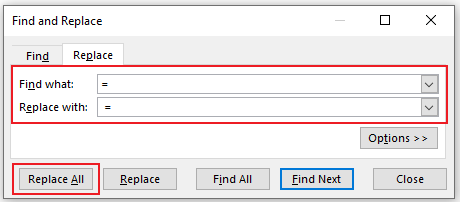##### Result

Now, a leading space has been added before all formulas in the selected cells, which results in the formulas being displayed as text.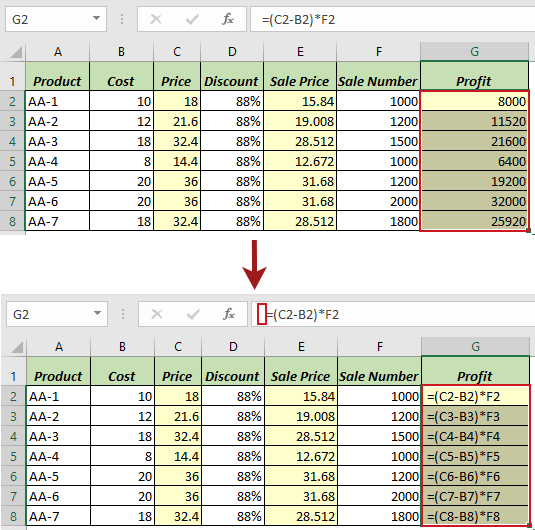Tips:
• To hide formulas and show results in the selected cells, in the Find and Replace dialog, input  = in the Find what box and input = in the Replace with box.
• In addition to replacing = with  = to display formulas, you can also replace = with '= (an apostrophe followed by the equal sign) to show them as text.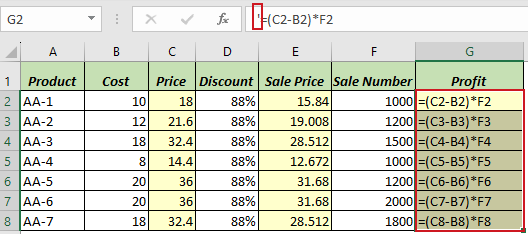This method adds a leading apostrophe to all formulas, making them appear as text. Note that the apostrophe will only be visible in the formula bar when the cell is selected; otherwise, it remains hidden.
• Even this approach is popular, but we don't recommend it because it has a limitation: you can't easily remove the leading apostrophe using the Find and Replace function to hide formulas and show results. To be specific, if you attempt to replace '= with = within the Find and Replace dialog, Excel will issue a warning indicating that no replacements could be made due to the absence of matching content.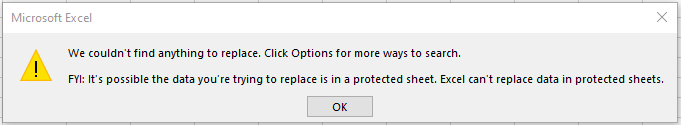### Why is Excel displaying the formula instead of the result?

Have you ever encountered a situation where, after entering a formula in a cell and pressing the Enter key, Excel stubbornly displays the formula instead of the result? Don't be alarmed; your Excel application is working correctly. We'll guide you through resolving this issue in no time.

If Excel shows a formula in a cell rather than its result, it might be caused by the following reasons:

##### Reason 1: Show Formulas Mode Activated

You may have unintentionally enabled the Show Formulas mode, either by clicking the Show Formulas button on the ribbon or by using the Ctrl + `shortcut.Solution:

To show the result, all you need to do is de-activate the Show Formulas mode, either by clicking the Show Formulas button on the ribbon again or by pressing the Ctrl + ` shortcut again.

If a formula starts with a leading space or an apostrophe ('), Excel treats it as text and will not perform any calculations it may contain.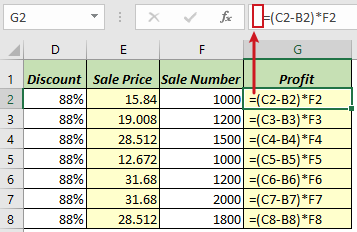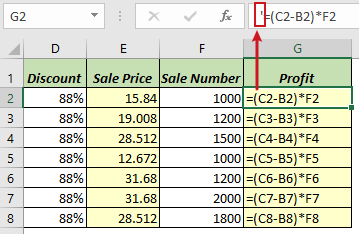Solution:

To show the result, all you need to do is remove the leading space or apostrophe before the equal sign in the formula.

##### Reason 3: Cell formatted as Text

If you accidentally set the cell's formatting to Text before inputting the formula in the cell, Excel also treats the formula as text and refrains from processing any formulas it contains.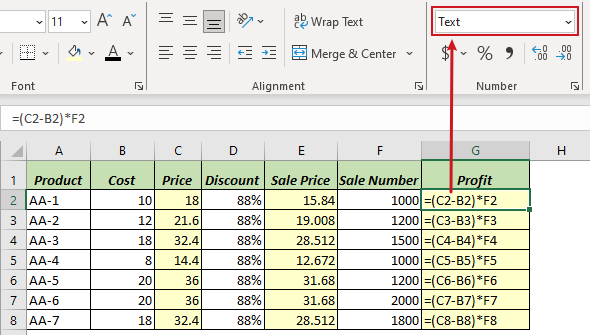Solution:

To show the result, select the relevant cell, then go to the Home tab and choose General from the Number group as the cell's format. After setting the format, double-click the cell or press the F2 key, and then hit Enter key.

Equipped with these detailed techniques, navigating and auditing your Excel sheets will become significantly more straightforward. If you're looking to explore more Excel tips and tricks, please click here to access our extensive collection of over thousands of tutorials.